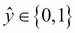# Classification with logistic regression

In the previous chapter, we saw how linear regression produces a predicted value, ŷ, from an input vector x and a vector of coefficients β:Here, ŷ can be any real number. Logistic regression proceeds in a very similar way, but adjusts the prediction to guarantee an answer only between zero and one:Zero and one represent two different classes. The change is a simple one; we simply wrap the prediction in a function g that constrains the output between zero and one:

Where g is called the sigmoid function. This seemingly ...

Get Clojure for Data Science now with the O’Reilly learning platform.

O’Reilly members experience live online training, plus books, videos, and digital content from nearly 200 publishers.